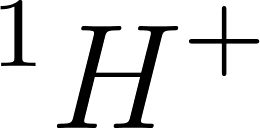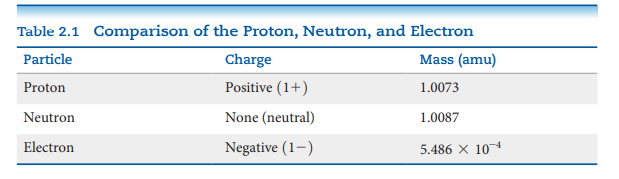×
Get Full Access to Chemistry: The Central Science - 13 Edition - Chapter 22 - Problem 3e
Get Full Access to Chemistry: The Central Science - 13 Edition - Chapter 22 - Problem 3e

×

# ?3EVisualizing ConceptsWhich of the following speciesISBN: 9780321910417 77

## Solution for problem 3E Chapter 22

Chemistry: The Central Science | 13th Edition

• Textbook Solutions
• 2901 Step-by-step solutions solved by professors and subject experts
• Get 24/7 help from StudySoup virtual teaching assistantsChemistry: The Central Science | 13th Edition

4 5 1 410 Reviews
12
2
Problem 3E

It is common in mass spectrometry to assume that the mass of a cation is the same as that of its parent atom. (a) Using data in Table 2.1, determine the number of significant figures that must be reported before the difference in masses ofandis significant. (b) What percentage of the mass of anatom does the electron represent?Step-by-Step Solution:

Step 1 of 3

(a)

The given isotopes -and. Those differ by only one proton.

According to the given table, the mass of the proton is 1.0073 amu.

Step 2 of 3

Step 3 of 3

##### ISBN: 9780321910417

Unlock Textbook Solution